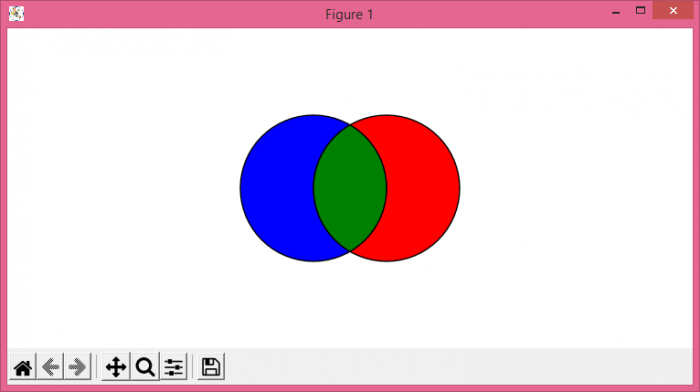# Coloring the Intersection of Circles/Patches in Matplotlib

To color the intersection of circles/patches in Matplotlib, we can take the following steps −

• Set the figure size and adjust the padding between and around the subplots.
• Create a and b points.
• Get the left, right and middle area from the two points, a and b.
• Get the current axes using gca() method
• Add patches with different colors and sections.
• Set the X and Y axes scale.
• Set the aspect ratios equal.
• Turn off the axes.
• To display the figure, use show() method.

## Example

import shapely.geometry as sg
import matplotlib.pyplot as plt
import descartes

plt.rcParams["figure.figsize"] = [7.50, 3.50]
plt.rcParams["figure.autolayout"] = True

a = sg.Point(-.5, 0).buffer(1.)
b = sg.Point(0.5, 0).buffer(1.)

left = a.difference(b)
right = b.difference(a)
middle = a.intersection(b)

ax = plt.gca()

ax.set_xlim(-2, 2)

ax.set_ylim(-2, 2)

ax.set_aspect('equal')

ax.axis('off')

plt.show()

## OutputUpdated on: 07-Jul-2021

327 Views